## Example Questions

### Example Question #1061 : Gre Quantitative Reasoningandare positive integers.is a multiple of.

Quantity A: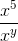Quantity B: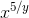Quantity A is greater.

The relationship cannot be determined.

The two quantities are equal.

Quantity B is greater.

The relationship cannot be determined.

Explanation:

Recall that the exponent of the denominator is sutracted from the exponent of the numerator.

Therefore Quantity A is equivalent to x5–y, and because we would then be comparing an arithmetic operation to a geometric operation, it does not matter whether y is a multiple of 5.  The two quantities cannot be compared.

### Example Question #3 : Mixed / Improper Fractions

Which of the following is the mixed fraction equivalent to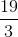?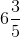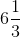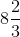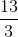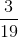Explanation:

To begin, notice that using your calculator, you can find: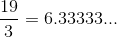Now, the closest even multiple ofthat is less thanis.  Therefore, you know that your number is: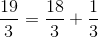This is the same as: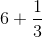, or simply,.  This is your mixed fraction.

### Example Question #1 : How To Find Out A Mixed Fraction From An Improper Fraction

Which of the following is equivalent to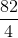?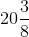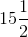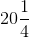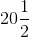Explanation:

Although there are many ways to convert improper fractions into mixed fractions, the easiest way is to use your calculator to your advantage.  Begin by dividing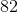by.  This gives you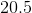. Therefore, you can eliminate all the options that have do not havefor their first portion. Next, multiplyby the denominator (), and get.  This means that you haveand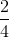, or.  Thus, your answer is.

### Example Question #2 : How To Find Out A Mixed Fraction From An Improper Fraction

Quantity A: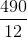Quantity B: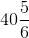Which of the following is true?

Quantity A is larger.

Quantity B is larger.

The two quantities are equal

The relationship of the two quantities cannot be determined based on the information provided.

Though there are several ways you could solve this, let's convert the improper fraction into a mixed one so we can compare them. Start by dividing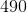by.  This gives you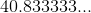Now, since we know that the two numbers have the same whole-number value, we need to compare their decimal portions. Compare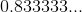to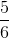. The latter is indeedTherefore, the two values are equal.